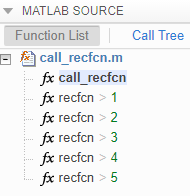## Avoid Duplicate Functions in Generated Code

### Issue

You generate code and it contains multiple, duplicate copies of the same functions, with only slight differences, such as modifications to the function signature. For example, your generated code might contain functions called `foo` and `b_foo`. Duplicate functions can make the generated code more difficult to analyze and manage.

### Cause

Duplicate functions in the generated code are the result of function specializations. The code generator specializes functions when it detects that they differ at different call sites by:

• Number of input or output variables.

• Type of input or output variables.

• Size of input or output variables.

• Values of input variables.

In some cases, these specializations are necessary for the generated C/C++ code because C/C++ functions do not have the same flexibility as MATLAB® functions. In other cases, the code generator specializes functions to optimize the generated code or because of a lack of information.

### Solution

In certain cases, you can alter your MATLAB code to avoid the generation of duplicate functions.

#### Identify Duplicate Functions by Using Code Generation Report

You can determine whether the code generator created duplicate functions by inspecting the code generation report or in Simulink®, the MATLAB Function report. The report shows a list of the duplicate functions underneath the entry-point function. For example:#### Duplicate Functions Generated for Multiple Input Sizes

If your MATLAB code calls a function multiple times and passes inputs of different sizes, the code generator can create specializations of the function for each size. To avoid this issue, use `coder.ignoreSize` on the function input. For example, this code uses `coder.ignoreSize` to avoid creating multiple copies of the function `indexOf`:

```function [out1, out2] = test1(in) a = 1:10; b = 2:40; % Without coder.ignoreSize duplicate functions are generated out1 = indexOf(coder.ignoreSize(a), in); out2 = indexOf(coder.ignoreSize(b), in); end function index = indexOf(array, value) coder.inline('never'); for i = 1:numel(array) if array(i) == value index = i; return end end index = -1; return end ```

To generate code, enter:

```codegen test1 -config:lib -report -args {1} ```

#### Duplicate Functions Generated for Different Input Values

If your MATLAB code calls a function and passes multiple different constant inputs, the code generator can create specializations of the function for each different constant. In this case, use `coder.ignoreConst` to indicate to the code generator not to treat the value as an immutable constant. For example:

```function [out3, out4] = test2(in) c = ['a', 'b', 'c']; if in > 0 c(2)='d'; end out3 = indexOf(c, coder.ignoreConst('a')); out4 = indexOf(c, coder.ignoreConst('b')); end function index = indexOf(array, value) coder.inline('never'); for i = 1:numel(array) if array(i) == value index = i; return end end index = -1; return end ```

To generate code, enter:

```codegen test2 -config:lib -report -args {1} ```

#### Duplicate Functions Generated for Different Number of Outputs

If your MATLAB code calls a function and accepts a different number of outputs at different call sites, the code generator can produce specializations for each call. For example:

```[a b] = foo(); c = foo(); ```

To make each call return the same number of outputs and avoid duplicate functions, use the `~` symbol:

```[a b] = foo(); [c, ~] = foo(); ```